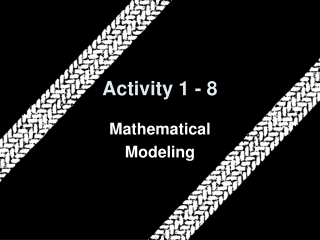DownloadDownload PresentationActivity 1 - 8

# Activity 1 - 8

Télécharger la présentation## Activity 1 - 8

- - - - - - - - - - - - - - - - - - - - - - - - - - - E N D - - - - - - - - - - - - - - - - - - - - - - - - - - -
##### Presentation Transcript

1. Activity 1 - 8 Mathematical Modeling

2. 5-Minute Check on Activity 1-7 • In functional notation, what is the input variable? • What are the restrictions on the independent variable? • In function notation, what is the output variable? • What are the restrictions on the dependent variable? • What is the domain and range of ? Domain (x-values and independent variable) no division by zero and no negatives under the radical Range (y-values and the dependent variable) dependent of functional definition 3 ---------- x - 2 Domain: x > 2 Range: y > 0 (graph it! we will see just how this works later) Click the mouse button or press the Space Bar to display the answers.

3. Objectives • Identify a mathematical model • Solve problems using formulas as models • Develop a function model to solve a problem • Recognize patterns and trends between two variables using tables as models

4. Vocabulary • Mathematical Model – is a mathematical form, such as a formula, an equation, a graph or table, that fits or approximates the important features of a given situation • Mathematical modeling – is the process of developing a mathematical model for a given situation

5. Activity After an automobile accident the investigating officers often estimate the speed of the vehicle by measuring the length of the tire skid distance. The following table gives the average skid distances for an automobile with good tires on dry pavement. Define the independent and dependent variables Does it appear to be a function?

6. y Activity Graph it x

7. Using our Calculator Following these instructions, graph the function • Put x data into L1 (STAT key , EDIT option) • Put y data into L2 • Hit 2nd key and then Y= (to get to stat plots) • Turn plot one on • Select the first graph • Graph by hitting ZOOM key and then option 9

8. Basal Energy Rate Basal energy rate is the daily amount of energy (in calories) needed by the body at rest to maintain body temperature and basic life processes. Basal energy differs for individuals, depending on their gender, age, height, and weight. The formula for the basal energy for men is: B = 655.096 + 9.563W + 1.85H – 4.676A where B is the basal rate, W is the weight (in kg), H is the height (in cm) and A is age (in years). • A male patient is 70 years old, weighs 55 kg and is 172 cm tall. Is a total daily caloric intake of 1000 calories enough to keep him properly fed? • A man is 178 cm tall and weighs 84 kg. If his basal energy rate is 1500 calories, how old is the man? B = 655.096 + 9.563(55) + 1.85(172) – 4.676(70) = 1171.941 cal needed 1500 = 655.096 + 9.563(84) + 1.85(178) – 4.676(A) about 61.5 years old

9. Windchill Table

10. Windchill Examine the windchill table on page 72 and answer the following questions: • What is the formula used to derive the values in the table? • What is the windchill for an air temperature of -30°F with a 20-mph wind? • If the wind speed is 40 mph, what is the windchill temperature if the air temperature is -20°F? • What is the windchill when the temperature is 5°F with a 25-mph wind? Wind Chill = 35.74 + 0.6215T – 35.75(V0.16) + 0.4275T(V0.16) Wind Chill = 35.74 + 0.6215(-30) – 35.75((20)0.16) + 0.4275(-30)((20)0.16) = -61.35 From the table: -57 From the table: -17

11. Roller Rink As part of a community service project at your high school, you organize a fund-raiser at the neighborhood roller rink. Money raised will benefit a summer camp for children with special needs. The admission charge is \$4.50 per person, \$2 of which is used to pay the rink’s rental fee. The remainder is donated. • What are the independent and dependent variables? • Write an equation with x representing the number attending and y as the amount donated. • If the maximum capacity of the rink is 200 people, what is the most that can be raised? Independent: number of people attending dependent: money raised y = f(x) = (4.50 – 2)x = 2.5x y = f(200) = 2.5(200) = 500 dollars

12. Summary and Homework • Summary • A mathematical model is a mathematical form, like an equation, formula, graph or table that fits or approximates important features of a given situation • Mathematical models can be simple or very complex • Mathematic modeling is the process of developing a mathematical model for a given situation • Homework • pg 74-7; 1-5